xTriad MethodEncyclopedia
The Triad Method, also known as the algebraic method, is a method for calculating the inclination of an object based on two known vectors
Vector (mathematics and physics)
In mathematics and physics, a vector is an element of a vector space. If n is a non negative integer and K is either the field of the real numbers or the field of the complex number, then K^n is naturally endowed with a structure of vector space, where K^n is the set of the ordered sequences of n...

in relation to that object's axis system. It was developed in 1964 by Harold D. Black and first published in the Journal of the American Institute of Aeronautics and Astronautics. The method was first devised when the first 3-axis solar panels where created. The panels allowed a satellite to know the direction of the sun thus combined with a vector of the direction of the earth, it was possible to determine the attitude
Attitude dynamics and control
Spacecraft flight dynamics is the science of space vehicle performance, stability, and control. It requires analysis of the six degrees of freedom of the vehicle's flight, which are similar to those of aircraft: translation in three dimensional axes; and its orientation about the vehicle's center...

of a satellite.

## The Triad Algorithm

The Triad algorithm takes as its input two pairs of reference vectors. Each pair of reference vectors represents some measured direction in both reference frames. For example, the first pair of reference vectors might be the measured direction of the sun in both the device frame of reference and the ECEF reference frame.

Three rotation matrices will be created. Using the reference vectors measured in the device frame, we will create a rotation matrix,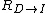, that rotates vectors from the device frame into the intermediate frame. We will then create a second rotation matrix,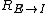, that rotates vectors from the ECEF frame into the intermediate frame. Finally, we will use these two intermediate rotation matrices to create a third rotation matrix,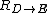, that converts vectors from the device frame directly into the ECEF frame.

The Triad algorithm itself is relatively straightforward and is divided into three steps:
1. Obtaining the reference vectors.
2. Creating the intermediate reference frame.
3. Solving for a full attitude solution.

### Obtaining the Reference Vectors

Before we can begin, we must obtain two pairs of corresponding reference vectors. For each reference frame, we will use as an example the measured magnetic field of the earth,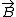, and the measured gravity vector,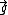. For the device reference frame, these will be denoted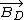and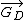, respectively, with the corresponding ECEF equivalents denoted by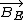and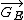.

### Creating the Intermediate Reference Frame

Once both pairs of reference vectors are obtained, we will use them to create an intermediate reference frame that is common to both the device and ECEF reference frames. We will use the reference vectors in each frame to create a rotation matrix into this intermediate frame, I. This intermediate reference frame will be created using the relative orientations of the reference vectors. Because these relative orientations are the same in every reference frame, the intermediate frame will be the same regardless of which reference frame we create it from.

To define the intermediate frame, we must create three orthonormal axes (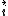,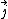, and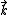) from the reference vectors in both the device and ECEF frames: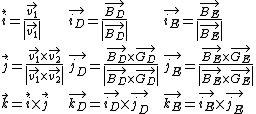NOTE: The choice offorwas arbitrary here, but for reasons that are beyond the scope of this article, the more accurate reference vector should always be chosen as.

We then combine these three basis vectors to create our intermediate rotation matrices: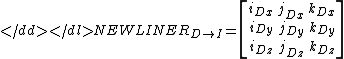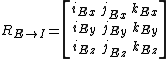### Solving for a Full Attitude Solution

We now have two rotation matrices,and, that rotate from each reference frame into the intermediate frame. To complete the algorithm and find a full attitude solution, we must define a sequence of rotations that takes us from the device frame to the intermediate frame and then finally to the ECEF frame. The first half of this sequence is accomplished by, but the second rotation matrix,, is the inverse of what we want.

To obtain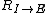, we can simply take the transpose: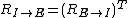Because the matrices and represent rotations, we can combine them in sequence by multiplying them together: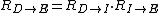This final rotation matrix represents the full attitude solution. We can now freely convert vector observations made in the device frame to the standard ECEF frame and back again: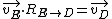## See also

• Attitude Dynamics and Control
Attitude dynamics and control
Spacecraft flight dynamics is the science of space vehicle performance, stability, and control. It requires analysis of the six degrees of freedom of the vehicle's flight, which are similar to those of aircraft: translation in three dimensional axes; and its orientation about the vehicle's center...

• Orientation (Geometry)
Orientation (geometry)
In geometry the orientation, angular position, or attitude of an object such as a line, plane or rigid body is part of the description of how it is placed in the space it is in....

• Wahba's problem
Wahba's problem
In applied mathematics, Wahba's problem, first posed by Grace Wahba in 1965, seeks to find a rotation matrix between two coordinate systems from a set of vector observations. Solutions to Wahba's problem are often used in satellite attitude determination utilising sensors such as magnetometers...

The source of this article is wikipedia, the free encyclopedia.  The text of this article is licensed under the GFDL.